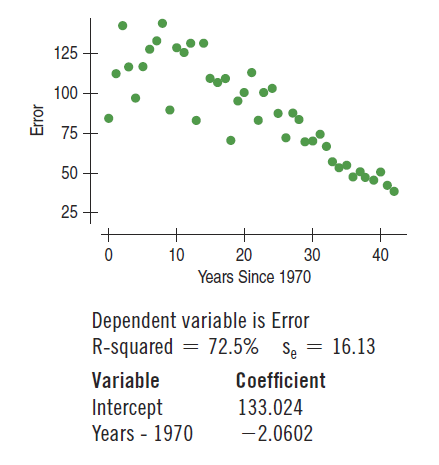×
Get Full Access to Stats: Data And Models - 4 Edition - Chapter 8 - Problem 24e
Get Full Access to Stats: Data And Models - 4 Edition - Chapter 8 - Problem 24e

×

# Tracking hurricanes 2012 In a previous chapter, we sawISBN: 9780321986498 70

## Solution for problem 24E Chapter 8

Stats: Data and Models | 4th Edition

• Textbook Solutions
• 2901 Step-by-step solutions solved by professors and subject experts
• Get 24/7 help from StudySoup virtual teaching assistantsStats: Data and Models | 4th Edition

4 5 1 238 Reviews
14
1
Problem 24E

Problem 24E

Tracking hurricanes 2012 In a previous chapter, we saw data on the errors (in nautical miles) made by the National Hurricane Center in predicting the path of hurricanes. The scatterplot at the top of the next page shows the trend in the 24-hour tracking errors since 1970 (www.nhc.noaa.gov).a) Interpret the slope and intercept of the model.

b) Interpret se in this context.

c) The Center would like to achieve an average tracking error of 45 nautical miles by 2015. Will they make it? Defend your response.

d) What if their goal were an average tracking error of 25 nautical miles?

Step-by-Step Solution:

Problem 24E

Tracking hurricanes 2012 In a previous chapter, we saw data on the errors (in nautical miles) made by the National Hurricane Center in predicting the path of hurricanes. The scatterplot at the top of the next page shows the trend in the 24-hour tracking errors since 1970 (www.nhc.noaa.gov).a) Interpret the slope and intercept of the model.

b) Interpret se in this context.

c) The Center would like to achieve an average tracking error of 45 nautical miles by 2015. Will they make it? Defend your response.

d) What if their goal were an average tracking error of 25 nautical miles?

Step by Step Solution

Step 1 of 5

(a)

The regression model can be written as :This is in the linear model form,  ie;where the b’s are called the coefficients of the linear model. The coefficientis the slope, which tells how rapidlychanges with respect to. The coefficientis the intercept, which tells where the line hits (intercepts) the y-axis.

Therefore,

The slope,= -2.0602, means error decreases on average of 2.0602 nautical miles per year. The intercept,= 133.024, indicates that the prediction error is 133.024 nautical miles in the year 1970.

Step 2 of 5

Step 3 of 5

##### ISBN: 9780321986498

Unlock Textbook Solution